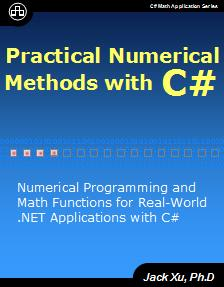## Practical Numerical Methods with C#

### Practical Numerical Methods with C#

###### You can order paperback book from Amazon

This book presents an in-depth exposition of the various numerical methods used in real-world scientific and engineering computations. It emphasizes the practical aspects of C# programming and numerical methods and mathematical functions. The book discusses various techniques that enable you to implement these numerical methods in your .NET applications. Click here for more information.#### eBook: \$39.99This book provides an in-depth exposition of the various numerical methods used in real-world scientific and engineering computations. It emphasizes the practical aspects of C# numerical methods and mathematical functions programming, and discusses in detail various techniques that will enable you to implement these numerical methods in your own .NET applications.

Ideal for scientists, engineers, and students who would like to become more adept with numerical methods, Practical Numerical Methods with C# familiarizes you with:

• The basics of C# programming.
• The mathematical background and fundamentals of numerical methods.
• Math libraries for complex numbers and functions, real and complex vector and matrix operations, and special functions.
• Numerical methods for generating random numbers and random distribution functions.
• Various numerical methods for solving linear and nonlinear equations.
• Numerical differentiation and integration.
• Interpolations and curve fitting.
• Optimization of single-variable and multi-variable functions using a variety of techniques, including advanced simulated annealing and evolutionary algorithms.
• Numerical techniques for solving ordinary differential equations.
• Numerical methods for solving boundary value problems.
• Eigenvalue problems.

In addition, this book provides code examples for every math function and numerical method to show you how to use these functions and methods in your own .NET applications.